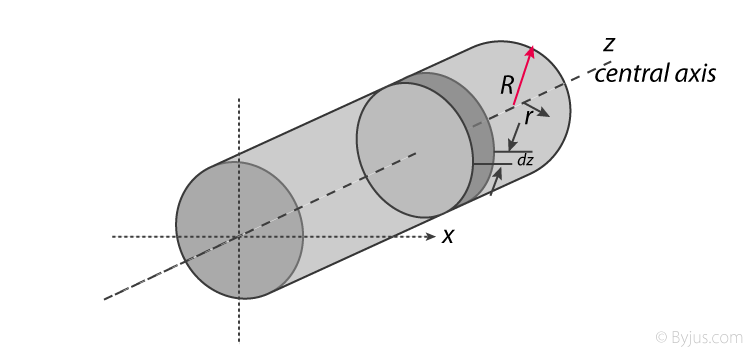Checkout JEE MAINS 2022 Question Paper Analysis : Checkout JEE MAINS 2022 Question Paper Analysis :

# Moment Of Inertia Of A Cylinder

Moment of inertia of cylinder expression is given here to help students know the right formulas for solving different problems based on the concept. In this lesson, they will find the moment of inertia equations for both solid and hollow cylinders.

## Solid Cylinder

A solid cylinder’s moment of inertia can be determined using the following formula;

 I = ½ MR2

Here, M = total mass and R = radius of the cylinder and the axis is about its centre.

To understand the full derivation of the equation for solid cylinder students can follow the interlink.

## Hollow Cylinder

The moment of inertia of a hollow cylinder rotating about an axis passing through the centre of the cylinder can be determined by the given formula;

 I = ½ M (R22 + R12)

Here, the cylinder will consist of an internal radius R1 and external radius R2 with mass M.

A hollow cylinder with a thin wall can be expressed using the following equation of moment of inertia;

 I = MR2

In this case, the axis is passing through the centre of the cylinder. M = mass and R = radius.

Students can learn the derivation of the hollow cylinder expression by following the link.

## Moment Of Inertia Of Cylinder About Perpendicular Axis

The moment of inertia of cylinder about a perpendicular axis passing through its centre is determined by;

Ix = ¼ (MR2 )+ 1/3( ML2)We will look at the derivation of this formula below.

Generally, the derivation involves 3 primary steps. It includes;

• Splitting the cylinder into infinitesimally thin disks and stating the moment of inertia.
• Using both parallel and perpendicular axis theorems to determine the expression.
• Integrating over the length of the cylinder.

1. Cutting the cylinder into infinitesimally thin disks

We will consider the cylinder having mass M, radius R, length L and the z-axis which passes through the central axis.

Here,

Density ρ = M / V

Next, we will consider the moment of inertia of the infinitesimally thin disks with thickness dz.

First, we assume that dm is the mass of each disk, We get;

dm = ρ x Volume of disk

dm = (M / V) x (πr2.dz)

We take V = area of circular face x length which is ( πr2L).

Now we obtain;

dm = (M / πr2L) x (πr2.dz)

dm = (M / L) dz

The moment of inertia about the central axis is given as;

dlz = ½ dmR2

2. Use of Perpendicular Axis Theorem

We now apply the perpendicular axis theorem which gives us;

dlz = dlx + dly

Here, if we need to consider that both x and y moments of inertia are equal by symmetry.

dlx = dly

We need to combine the equations for the perpendicular axis theorem and symmetry. We get;

dlx = dlz / 2

Now we substitute lz from the equation above.

dlx = ½ x [½ dmR2]

dlx = ¼ dmR2

Alternatively, for the x-axis, we use the parallel axis theorem to find the moment of inertia. We get;

dlx = ¼ dmR2 + dmz2

3. Integration

Now we conduct integration over the length of the cylinder to express the mass element dm in terms of z. We take the integral from z=0 to z=L.

Ix = oL dlx

Ix = oL ¼  (M / L) R2 dz+ oL z (M / L)dz

Ix = [¼ (M / L) R2z + (M / L) z3 / 3]0L

Since it is a definite form of integral we ignore the constant. We will now have;

Ix = ¼ (M / L) R2 L + ML3 / 3

Ix = MR2 /4+ ML2/3

## Parallel Axis TheoremTest Your Knowledge On Moment Of Inertia Of A Cylinder!